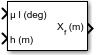# LLA to ECEF Position

Calculate Earth-centered Earth-fixed (ECEF) position from geodetic latitude, longitude, and altitude above planetary ellipsoid

•Libraries:
Aerospace Blockset / Utilities / Axes Transformations

## Description

The LLA to ECEF Position block converts geodetic latitude $\left(\overline{\mu }\right)$, longitude $\left(\overline{\iota }\right)$, and altitude $\left(\overline{h}\right)$ above the planetary ellipsoid into a 3-by-1 vector of ECEF position $\left(\overline{p}\right)$. Latitude and longitude values can be any value. However, latitude values of +90 and -90 may return unexpected values because of singularity at the poles. For more information the ECEF position calculation, see Algorithms.

## Limitations

• The planet is assumed to be ellipsoidal. To use a spherical planet, set the Flattening parameter to zero.

• The implementation of the ECEF coordinate system assumes that the origin is at the center of the planet, the x-axis intersects the Greenwich meridian and the equator, the z-axis is the mean spin axis of the planet, positive to the north, and the y-axis completes the right-handed system.

## Ports

### Input

expand all

Geodetic latitude and longitude, specified as a 2-by-1 vector, in degrees.

Data Types: `double`

Altitude above the planetary ellipsoid, specified as a scalar.

Data Types: `double`

### Output

expand all

Position in ECEF frame, returned as a 3-by-1 vector, in the same units as the input at the h port.

Data Types: `double`

## Parameters

expand all

Parameter and output units:

Units

Radius from CG to Center of Planet

`Metric (MKS)`

Meters

Meters

`English`

Feet

Feet

#### Dependencies

To enable this, set Planet model to `Earth (WGS84)`.

#### Programmatic Use

 Block Parameter: `units` Type: character vector Values: ```'Metric (MKS)'``` | `'English'` Default: ```'Metric (MKS)'```

Planet model to use, `Custom` or `Earth (WGS84)`.

#### Programmatic Use

 Block Parameter: `ptype` Type: character vector Values: ```'Earth (WGS84)'``` | `'Custom'` Default: ```'Earth (WGS84)'```

Flattening of the planet, specified as a double scalar.

#### Dependencies

To enable this parameter, set Planet model to `Custom`.

#### Programmatic Use

 Block Parameter: `F` Type: character vector Values: double scalar Default: `1/298.257223563`

Radius of the planet at its equator, in the same units as the desired units for ECEF position.

#### Dependencies

To enable this parameter, set Planet model to `Custom`.

#### Programmatic Use

 Block Parameter: `R` Type: character vector Values: double scalar Default: `6378137`

## Algorithms

The ECEF position is calculated from the geocentric latitude at mean sea-level (λs) and longitude using:

`$\overline{p}=\left[\begin{array}{c}{\overline{p}}_{x}\\ {\overline{p}}_{y}\\ {\overline{p}}_{z}\end{array}\right]=\left[\begin{array}{c}{r}_{s}\mathrm{cos}{\lambda }_{s}\mathrm{cos}\iota +h\mathrm{cos}\mu \mathrm{cos}\iota \\ {r}_{s}\mathrm{cos}{\lambda }_{s}\mathrm{sin}\iota +h\mathrm{cos}\mu \mathrm{sin}\iota \\ {r}_{s}\mathrm{sin}{\lambda }_{s}+h\mathrm{sin}\mu \end{array}\right],$`

where geocentric latitude at mean sea-level and the radius at a surface point (rs) are defined by flattening $\left(\overline{f}\right)$, and equatorial radius $\left(\overline{R}\right)$ in the following relationships:

`$\begin{array}{l}{\lambda }_{s}=\text{atan}\left({\left(1-f\right)}^{2}\mathrm{tan}\mu \right)\\ \\ {r}_{s}=\sqrt{\frac{{R}^{2}}{1+\left(1/{\left(1-f\right)}^{2}-1\right){\mathrm{sin}}^{2}{\lambda }_{s}}}\end{array}$`

 Stevens, B. L., and F. L. Lewis. Aircraft Control and Simulation, Hoboken, NJ: John Wiley & Sons, 1992.

 Zipfel, Peter H., Modeling and Simulation of Aerospace Vehicle Dynamics. Second Edition. Reston, VA: AIAA Education Series, 2000.

 Recommended Practice for Atmospheric and Space Flight Vehicle Coordinate Systems, R-004-1992, ANSI/AIAA, February 1992.